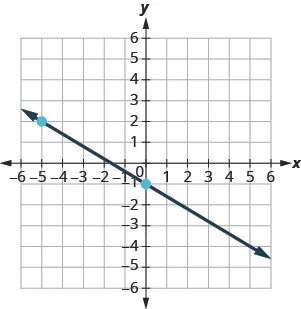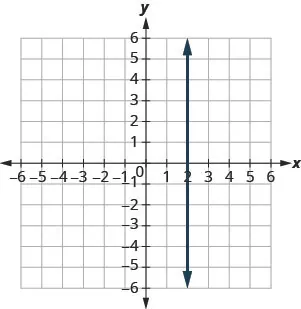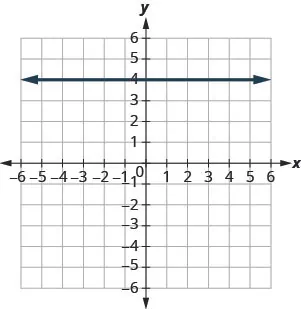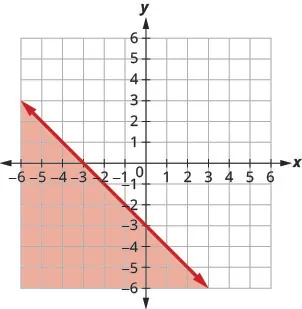Elementary Algebra

# Practice Test

Elementary AlgebraPractice Test

### Practice Test

682.

Plot each point in a rectangular coordinate system.

$(2,5)(2,5)$
$(−1,−3)(−1,−3)$
$(0,2)(0,2)$
$(−4,32)(−4,32)$
$(5,0)(5,0)$

683.

Which of the given ordered pairs are solutions to the equation $3x−y=63x−y=6$?

$(3,3)(3,3)$
$(2,0)(2,0)$
$(4,−6)(4,−6)$

684.

Find three solutions to the linear equation $y=−2x−4y=−2x−4$.

685.

Find the x- and y-intercepts of the equation $4x−3y=124x−3y=12$.

Find the slope of each line shown.

686.687.688.689.

Find the slope of the line between the points $(5,2)(5,2)$ and $(−1,−4)(−1,−4)$.

690.

Graph the line with slope $1212$ containing the point $(−3,−4)(−3,−4)$.

Graph the line for each of the following equations.

691.

$y = 5 3 x − 1 y = 5 3 x − 1$

692.

$y = − x y = − x$

693.

$x − y = 2 x − y = 2$

694.

$4 x + 2 y = −8 4 x + 2 y = −8$

695.

$y = 2 y = 2$

696.

$x = −3 x = −3$

Find the equation of each line. Write the equation in slope–intercept form.

697.

slope $−34−34$ and y-intercept $(0,−2)(0,−2)$

698.

$m=2m=2$, point $(−3,−1)(−3,−1)$

699.

containing $(10,1)(10,1)$ and $(6,−1)(6,−1)$

700.

parallel to the line $y=−23x−1y=−23x−1$, containing the point $(−3,8)(−3,8)$

701.

perpendicular to the line $y=54x+2y=54x+2$, containing the point $(−10,3)(−10,3)$

702.

Write the inequality shown by the graph with the boundary line $y=−x−3y=−x−3$.Graph each linear inequality.

703.

$y > 3 2 x + 5 y > 3 2 x + 5$

704.

$x − y ≥ −4 x − y ≥ −4$

705.

$y ≤ −5 x y ≤ −5 x$

706.

$y < 3 y < 3$

Order a print copy

As an Amazon Associate we earn from qualifying purchases.